# The MI Procedure

### Monotone and FCS Logistic Regression Methods

Subsections:

The logistic regression method is another imputation method available for classification variables. In the logistic regression method, a logistic regression model is fitted for a classification variable with a set of covariates constructed from the effects, where the classification variable is an ordinal response or a nominal response variable.

In the MI procedure, ordered values are assigned to response levels in ascending sorted order. If the response variable Y takes values in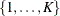, then for ordinal response models, the cumulative model has the form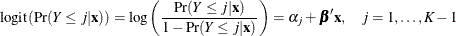where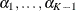are K-1 intercept parameters, and
is the vector of slope parameters.

For nominal response logistic models, where the K possible responses have no natural ordering, the generalized logit model has the form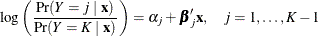where theare K-1 intercept parameters, and the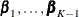are K-1 vectors of slope parameters.

#### Binary Response Logistic Regression

For a binary classification variable, based on the fitted regression model, a new logistic regression model is simulated from the posterior predictive distribution of the parameters and is used to impute the missing values for each variable (Rubin 1987, pp. 167–170).

For a binary variable Y with responses 1 and 2, a logistic regression model is fitted using observations with observed values for the imputed variable Y: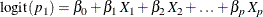where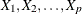are covariates for Y,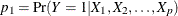,   and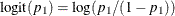The fitted model includes the regression parameter estimates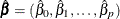and the associated covariance matrix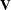.

The following steps are used to generate imputed values for a binary variable Y with responses 1 and 2:

1. New parameters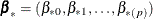are drawn from the posterior predictive distribution of the parameters.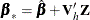where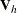is the upper triangular matrix in the Cholesky decomposition,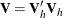, andis a vector of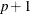independent random normal variates.

2. For an observation with missing
and covariates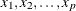, compute the predicted probability that Y= 1: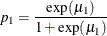where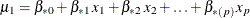.

3. Draw a random uniform variate, u, between 0 and 1. If the value of u is less than, impute Y= 1; otherwise impute Y= 2.

The binary logistic regression imputation method can be extended to include the ordinal classification variables with more than two levels of responses, and the nominal classification variables. The LINK=LOGIT and LINK=GLOGIT options can be used to specify the cumulative logit model and the generalized logit model, respectively. The options ORDER= and DESCENDING can be used to specify the sort order for the levels of the imputed variables.

#### Ordinal Response Logistic Regression

For an ordinal classification variable, based on the fitted regression model, a new logistic regression model is simulated from the posterior predictive distribution of the parameters and is used to impute the missing values for each variable.

For a variable Y with ordinal responses 1, 2, …, K, a logistic regression model is fitted using observations with observed values for the imputed variable Y: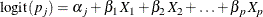whereare covariates for Y and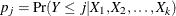.

The fitted model includes the regression parameter estimates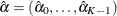and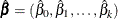, and their associated covariance matrix.

The following steps are used to generate imputed values for an ordinal classification variable Y with responses 1, 2, …, K:

1. New parameters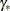are drawn from the posterior predictive distribution of the parameters.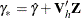where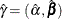,is the upper triangular matrix in the Cholesky decomposition,, andis a vector of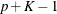independent random normal variates.

2. For an observation with missing Y and covariates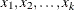, compute the predicted cumulative probability for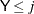: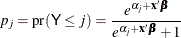3. Draw a random uniform variate, u, between 0 and 1, then impute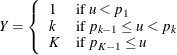#### Nominal Response Logistic Regression

For a nominal classification variable, based on the fitted regression model, a new logistic regression model is simulated from the posterior predictive distribution of the parameters and is used to impute the missing values for each variable.

For a variable Y with nominal responses 1, 2, …, K, a logistic regression model is fitted using observations with observed values for the imputed variable Y: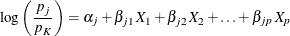whereare covariates for Y and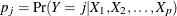.

The fitted model includes the regression parameter estimatesand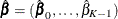, and their associated covariance matrix, where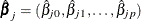,

The following steps are used to generate imputed values for a nominal classification variable Y with responses 1, 2, …, K:

1. New parametersare drawn from the posterior predictive distribution of the parameters.where,is the upper triangular matrix in the Cholesky decomposition,, andis a vector ofindependent random normal variates.

2. For an observation with missing Y and covariates, compute the predicted probability for Y= j, j=1, 2, …, K-1: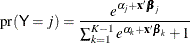and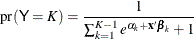3. Compute the cumulative probability for: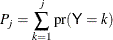4. Draw a random uniform variate, u, between 0 and 1, then impute#### Logistic Regression with Augmented Data

In a logistic regression model, you might not be able to find the maximum likelihood estimates of the parameters if there is no overlap of the sample points from response groups—that is, if the data points have either a complete separation pattern or a quasi-complete separation pattern.

Complete separation of data points occurs when a linear combination of predictors correctly allocates all observations to their response groups. Quasi-complete separation occurs when a linear combination of predictors correctly allocates all observations to their response groups except for a subset of observations where the values of linear combinations of predictors are identical. For more information about complete separation patterns and quasi-complete separation patterns, see the section Existence of Maximum Likelihood Estimates in Chapter 72: The LOGISTIC Procedure.

To address the separation issue in multiple imputation, White, Daniel, and Royston (2010) add observations to each response group and then use the augmented data to fit a weighted logistic regression. In each response group, 2p observations are added, where p is the number of predictors. More specifically, corresponding to each predictor, two observations are added: the first with the predictor mean minus the predictor standard deviation, and the second with the predictor mean plus the predictor standard deviation. In both observations, the values of other predictors are fixed at their corresponding means. Each additional observation contributes the same weight, and the total added weight is p+1. Each available observation in the data set (before augmentation) has a weight of 1. With this approach, there is an overlap of sample points, and maximum likelihood estimates can be obtained.

In the MONOTONE and FCS statements, the LIKELIHOOD=AUGMENT suboption in the LOGISTIC option requests maximum likelihood estimates based on augmented data. When LIKELIHOOD=AUGMENT, you can use the WEIGHT=w option to specify the total added weight w explicitly, or you can use the WEIGHT=NPARM option to specify the number of parameters as the total added weight. More specifically, for logistic regression models that consist only of p continuous effects, the added weight is p+1 for a simple binary logistic model, p+k–1 for an ordinal response model, and (p+1) (k–1) for a nominal response model, where k is the number of response levels.

If the ratio between the number of parameters and the number of available observations (before augmentation) is large, the effect from the added observations in the computation of maximum likelihood estimates can be significant. You can use the MULT=m suboption in the WEIGHT=NPARM option to reduce the total added weight, where the multiplier 0 < m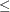1. The resulting total added weight is then m times the number of parameters. Alternatively, you can use the WEIGHT=w option to specify a smaller total added weight w explicitly.